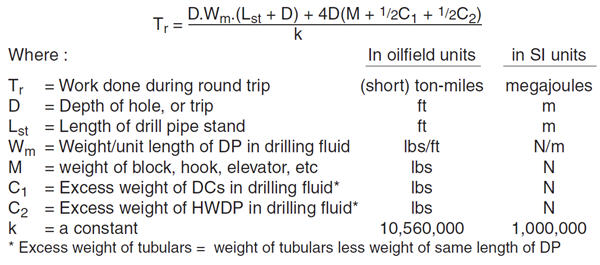# Round trip ton-miles Calculation

All types of ton-mile service should be calculated and recorded in order to obtain a true picture of the total service received from the rotary drilling line. There are several types of ton miles as follows;

1. Round trip ton-miles
2. Drilling or “connection” ton-miles
3. Coring ton-miles
4. Ton-miles setting casing
5. Short-trip ton-miles

For this time, I will show how to calculate round trip ton-mile.

Round Trip Ton-Miles CalculationThe formula for round trip ton-miles is listed below;

RTTM = (Wp x D x (Lp + D) + (2 x D) x (2 x Wb + Wc)) ÷ (5280 x 2000)

where
RTTM = Round Trip Ton-Miles
Wp = buoyed weight of drill pipe in lb/ft
D = hole measured depth in ft
Lp = Average length per stand of drill pipe in ft
Wb = weight of travelling block in lb
Wc = buoyed weight of BHA (drill collar + heavy weight drill pipe + BHA) in mud minus the buoyed weight of the same length of drill pipe in lb
** If you have BHA (mud motor, MWD, etc) and HWDP, you must add those weight into calculation as well not just only drill collar weight. **
2000 = number of pounds in one ton
5280 = number of feet in one mile

Note: One ton-mile equals 10,560,000 foot- pounds, and is equivalent to lifting 2,000 pounds a distance of 5,280 feet

Example: Round trip ton-miles

Mud weight = 10.0 ppg
Average length per stand = 94 ft
Drill pipe weight = 13.3 lb/ft
Hole measure depth = 5500 ft
Drill collar length = 120 ft
Drill collar weight = 85 lb/ft
HWDP length = 49 lb/ft
HWDP weight = 450 ft
BHA weight from directional driller = 8,300 lb
BHA length = 94 ft
Travelling block assembly = 95,000 lb

Solution:

a) Buoyancy factor:
BF = (65.5 – 10.0) ÷ 65.5
BF = 0.847

b) Buoyed weight of drill pipe in mud, lb/ft (Wp):
Wp = 13.3 lb/ft x 0.847
Wp = 11.27 lb/ft

c) buoyed weight of BHA (drill collar + heavy weight drill pipe + BHA) in mud minus the buoyed weight of the same length of drill pipe in lb (Wc):

Wc = {[(120×85) + (49×450) + (8300)] x 0.847} – [(120+450+94) x13.3x 0.847]
Wc = 26,866 lb

Round trip ton-miles = [(11.27 x 5500 x (94+ 5500)) + (2 x 5500) x (2 x 95000 + 26,866)] ÷ (5280 x 2000)
RTTM = 258.75 ton-mile

Share the joyWorking in the oil field and loving to share knowledge.

### 9 Responses to Round trip ton-miles Calculation

1.Henry Freites says:

Necesito por favor que me ayude con las formulas de toneladas millas
(Please I need help with formulas tons miles I appreciate it. I hope to answer.)

2.davin jackle says:

hey there. do you know how to convert ton-miles to megajoules?

•DrillingFormulas.Com says:

Hi Davin,

Please see the ton mile calculation in megajoules.Regards,
Shyne

3.tony says:

it is more than you can get on ton miles and make your cut

thanks

4.Patrick Tabone says:

Is this formula good for any drill line size? and does it apply for any number of lines in the derrick? If not what’s the formula for 2″ drill line with 16 lines in the derrick?

•DrillingFormulas.Com says:

This formula is a generic ton-mile for any line. You need to compare the ton-mile figure with the drilling line specification data from the manufacture to determine when you will cut the drilling line.

5.G.medok says:

Valuable and ezy for caculation.csn put up formulae on microsoft excel sheet too.

This site uses Akismet to reduce spam. Learn how your comment data is processed.